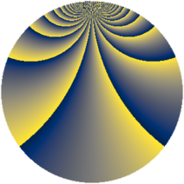# Properties

 Label 2240.2.dnLevel $2240$ Weight $2$ Character orbit 2240.dn Rep. character $\chi_{2240}(33,\cdot)$ Character field $\Q(\zeta_{12})$ Dimension $384$ Sturm bound $768$

# Related objects

## Defining parameters

 Level: $$N$$ $$=$$ $$2240 = 2^{6} \cdot 5 \cdot 7$$ Weight: $$k$$ $$=$$ $$2$$ Character orbit: $$[\chi]$$ $$=$$ 2240.dn (of order $$12$$ and degree $$4$$) Character conductor: $$\operatorname{cond}(\chi)$$ $$=$$ $$280$$ Character field: $$\Q(\zeta_{12})$$ Sturm bound: $$768$$

## Dimensions

The following table gives the dimensions of various subspaces of $$M_{2}(2240, [\chi])$$.

Total New Old
Modular forms 1632 384 1248
Cusp forms 1440 384 1056
Eisenstein series 192 0 192

## Trace form

 $$384q + O(q^{10})$$ $$384q + 288q^{81} + O(q^{100})$$

## Decomposition of $$S_{2}^{\mathrm{new}}(2240, [\chi])$$ into newform subspaces

The newforms in this space have not yet been added to the LMFDB.

## Decomposition of $$S_{2}^{\mathrm{old}}(2240, [\chi])$$ into lower level spaces

$$S_{2}^{\mathrm{old}}(2240, [\chi]) \cong$$ $$S_{2}^{\mathrm{new}}(280, [\chi])$$$$^{\oplus 4}$$$$\oplus$$$$S_{2}^{\mathrm{new}}(1120, [\chi])$$$$^{\oplus 2}$$